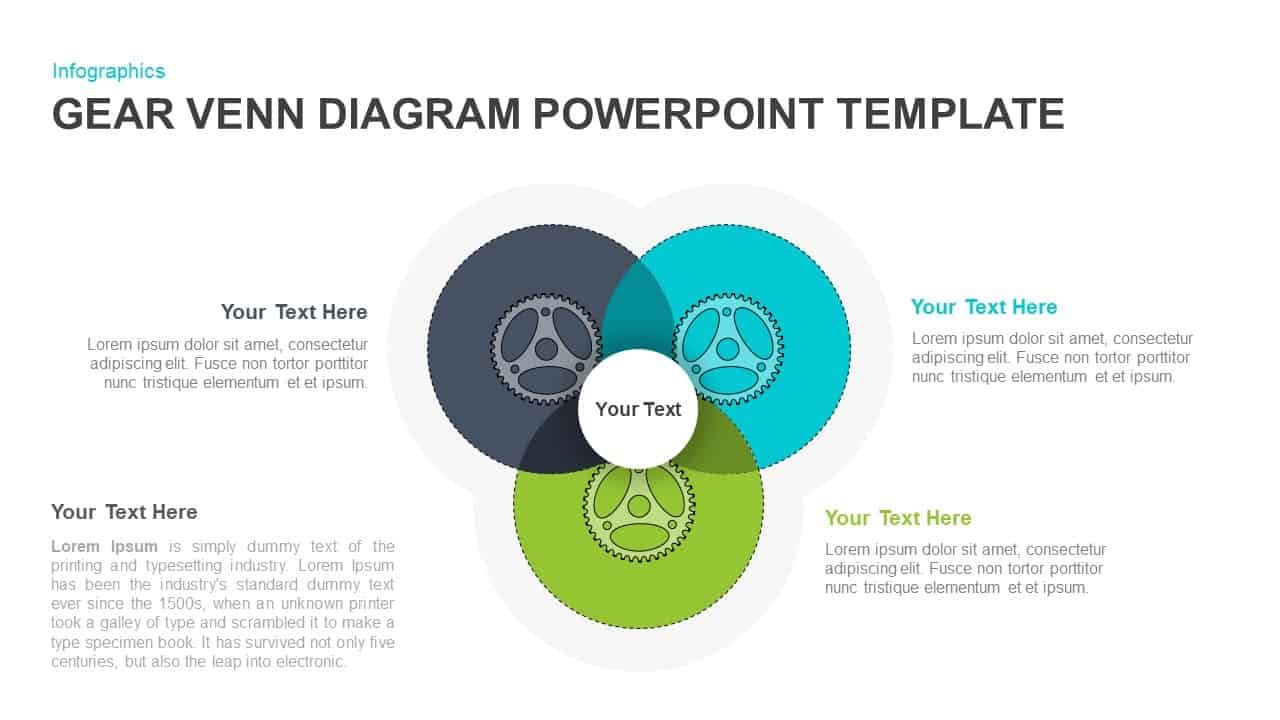••## Gear Venn Diagram Template for PowerPoint Presentation

Gear Venn diagram PowerPoint template shows the gear PowerPoint graphics on the intersecting circle wheels. The three interconnecting circles are representing three sets, ideally useful for showing logical relationships. This mathematical representation is an easy way to validate the logical relationship between seemingly unrelated ‘sets’ of different groups of things. This PowerPoint slide is perfect of any professional user looking to illustrate three interrelated subjects and the concomitant outcomes. Pictographic representation of sets represented by closed figures is called set diagrams or Venn diagrams. It can be used to illustrate various operations like a merger, intersection, and difference. The presenters can display the relationship among sets through this is in a more significant way. Venn diagram PowerPoint template is an easy to understand simple diagram model, it allows the spectator to visualize the overlap between variables in your data and also how they contrast. The presenter can easily show the logic behind his/her statements or equation and compare two or more data sets or mixtures.

The Venn diagram ppt template in gear shape shows the logical connections of the loop. This is an infinity diagram template that displays the never-ending process or the repetition of variables. The template is created with looping circles, which are colored distinctively for the easy drawing out the presentation topic. It is also a comparison chart, which demonstrates the common features of two or more sets. The teachers can use the Venn diagram to show the relationship of two or more items. For instance, what are the common features of a rectangle and a square? And they can put one more items in the sets and the question can be changed; what are the common features of rectangle, square and parallelogram? Hence, the gear-shaped Venn diagram ppt template is fit for any type of logical projections using scientific methods.

The Venn diagram PowerPoint template is a flat vector template of 2 slides. It offers text areas for all three segments. These allow the presenter to enter the appropriate textual content, key notes, and information about each ringing circle. The users can editable PowerPoint is fit for presenting mathematical relationships.

You can access more Venn Diagrams here Grab the free ppt now!